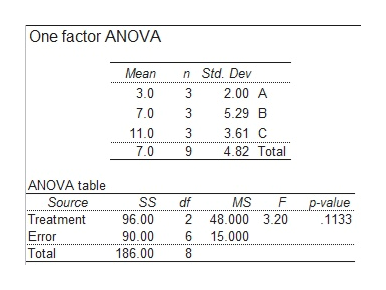# Calculate the ANOVA for the following data:Sample A      Sample B        Sample C            1                     3                    7  3                      5                  125                        13                 14State the Null and alternative hypotheses.  Calculate the F ratio. Is there a significant difference? Which groups are significantly different from each other?

Question
6 views

Calculate the ANOVA for the following data:

Sample A      Sample B        Sample C            1                     3                    7

3                      5                  12

5                        13                 14

State the Null and alternative hypotheses.  Calculate the F ratio. Is there a significant difference? Which groups are significantly different from each other?

check_circle

Step 1

Note:

Thank you for posting the question. We have considered the values after sample C as the first row and preceded the calculations.

Step 2

Null Hypothesis:

There is no significant difference among the means of three samples.

Alternative Hypothesis:

There is a significant difference among the means of three samples.

Enter the given data in EXCEL sheet as shown below:

Step 3

Step-by-step procedure to obtain ANOVA test statistic using MegaStat:

• Go to MegaStat > Analysis of variance > One-factor ANOVA.
• Select the input range of th...help_outlineImage TranscriptioncloseOne factor ANOVA Mean n Std. Dev 3.0 3 2.00 A 7.0 3 5.29 B 11.0 3 3.61 C 4.82 Total 7.0 9 ANOVA table Source p-value SS df MS Treatment 96.00 48.000 3.20 1133 Error 90.00 6 15.000 Total 186.00 8 NCOCO fullscreen

### Want to see the full answer?

See Solution

#### Want to see this answer and more?

Solutions are written by subject experts who are available 24/7. Questions are typically answered within 1 hour.*

See Solution
*Response times may vary by subject and question.
Tagged in

### Other